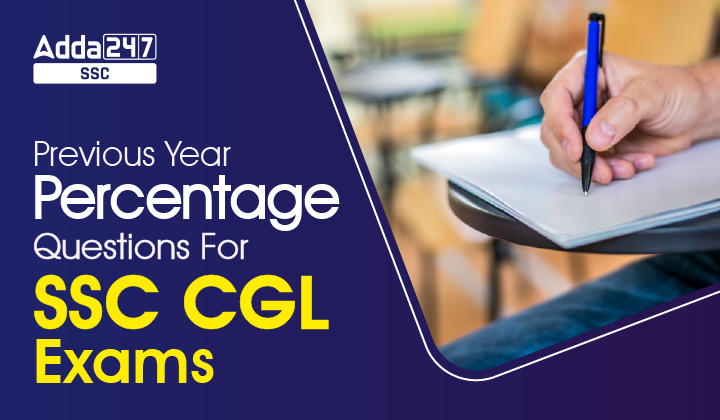Latest SSC jobs   »   SSC CGL   »   Previous Year Percentage Questions for SSC...

# Previous Year Percentage Questions for SSC CGL ExamsDear Students, SSC has released the notification for CGL. This time again the competition is going to be very stiff. Questions from Quant are asked in Tier-1 and Tier-II as well. Hence, you need to focus on this subject more. The only trick to master Quant is “practice‘. So, Practice daily. We are providing topic-wise quant quizzes, solve, learn, succeed.
Q1. Mr Amar spends 50% of his monthly income on household items and out of the remaining he spends 25% on travelling, 30% on entertainment, 15% on shopping and remaining amount of Rs. 900 is saved. What is Mr Amar’s monthly income?
(a) Rs. 6,000
(b) Rs. 12,000
(c) Rs. 9,000
(d) Cannot be determined
S1. Ans.(a)
Sol. House hold expenditure = 50%
Remaining = 50%
On Remaining amount he spends:-
Travelling = 25%
Entertainment = 30%
Shopping = 15%
Total = 70%  Remaining = (100 – 70) % of 50%
Rest amount  900 = 30% of 50%
15% = 900
100% = 6,000.
Q2. In a test consisting of 80 questions carrying one mark each, Ankita answers 65% of the first 40 questions correctly. What percent of the other 40 questions does she need to answer correctly to score 80% on the entire test?
(a) 60
(b) 80
(c) 95
(d) 40
S2. Ans.(c)
Sol. Total marks of test = 80
Ankita scored = 65% of 40 Question (1 mark)
= 26
But she needs to score = 80% of entire ‘test’
= 80% of 80
= 64 marks
So, she needs = (64 – 26) marks
= 38
Percentage ⇒ x% of 40 = 38
x = 95

Q3. In a class of 60 students and 5 teachers, each student got sweets that are 20% of the total number of students and each teacher got sweets that are 30% of the total number of students. How many sweets were there?
(a) 845
(b) 897
(c) 810
(d) 104
S3. Ans.(c)
Sol. No. of students = 60
No. of teachers = 5
No. of sweets each students got = 20% of (60)
= 12
Total sweets distributed among students
= 12 × 60 =720
No. of sweets each teacher got = 30% of (60)
= 18
Total sweets = 18 × 5 = 90
distributed among teachers
Total sweets = (720 + 90)
= 810
Q4. In a college election fought between two candidates, one candidate got 55% of the total valid votes. 15% of the votes were invalid. If the total votes were 15,200, what is the number of valid votes the other candidate got?
(a) 7106
(b) 6840
(c) 8360
(d) 5814
S4. Ans.(d)
Sol. Let total votes = 100%
Invalid = 15%
1st candidate = 55% of 85%
So, 2nd candidate = 45% of 85%
According to the question,
100%  15200
85%  12920
Other candidate got = 45% of 12920
= 5814 vote

Q5. On a test consisting of 250 questions, John answered 40% of the first 125 questions correctly. What percent of the other 125 questions does she need to answer correctly for her grade on the entire exam to be 60%?
(a) 75
(b) 80
(c) 60
(d) Cannot be determined
Q6. If the numerator of a fraction is increased by 400% and the denominator of the fraction is increased by 150%, the resultant fraction is 3/5. What is the original fraction?
(a) 3/10
(b) 1/8
(c) 3/7
(d) 2/7

Q7. In a two digit positive number, the digit in the units place is equal to the square of the digit in ten’s place, and the difference between the number obtained by interchanging the digits and the number is 54. What is 10% of the original number?
(a) 15.6
(b) 3.9
(c) 37.2
(d) 24
We’ll check the difference of two numbers
42 – 24 = 18 û
and
93 – 39 = 54 ü
So, number = 39
10% of 39 = 3.9

Q8. A candidate who scores 30% fails by 10 marks, while another candidate who scores 40% marks gets 15 more than minimum pass marks. The minimum marks required to pass are:
(a) 85
(b) 70
(c) 100
(d) 150

Q9. 65% of a number is more than its 25% by 120. What is 20% of that number?
(a) 66
(b) 69
(c) 48
(d) 60
S9. Ans.(d)
Sol. According to the question,
65% – 25% = 120
40% = 120
1% = 3
100% = 300
20% = 60

Q10. If the radius of a right circular cone is decreased by 50% and its height is increased by 50%, its volume will be decreased by:
(a) 10%
(b) 62.5%
(c) 40%
(d) 20%
Q12. The price of sugar is increased by 20%. As a result, a family has decreased its consumption by 30%. The expenditure of the family on sugar is decreased by:
(a) 0%
(b) 2.5%
(c) 16%
(d) 5%

Q13. If the income tax is increased by 19% the net income is reduced by 1%. The rate of income tax is:
(a) 6%
(b) 4%
(c) 5%
(d) 7.2%
Q14. The population of a village was 9600. In a year, with the increase in the population of males by 8% and that of females by 5%, the population of the village became 10176. What was the number of males in the village before increase?
(a) 4200
(b) 4410
(c) 3200
(d) 6400

Q15. If 60% of the students in a school are boys and the number of girls is 972, how many boys are there in the school?
(a) 1258
(b) 1458
(c) 1324
(d) 1624

## FAQs

### Is SSC CGL Notification 2023 released?

As per the SSC Calendar 2023-24, the SSC CGL Notification 2023 will be released on 1st April 2023.